# Correlation Matrix In ExcelArticle byExcelMojo Team## What Is Correlation Matrix In Excel?

The Correlation Matrix in Excel is a square and symmetrical table containing the correlation coefficients of the specified variables. And the matrix helps analyze the degree of the linear relationship between all the possible variable pairs.

Users can use the Excel Correlation Matrix to summarize a massive dataset and determine correlation patterns to make decisions accordingly.

For example, the table below contains the values of two variables in a dataset.

To calculate Correlation Matrix in Excel to analyze the relationship pattern between the two specified variables, we can use the Correlation analysis tool in the Analysis ToolPak feature in the Data tab to achieve the required correlation matrix.

The above Correlation Coefficient Matrix Excel shows that the correlation coefficient for the variables X and Y is 0.61316, indicating a positive correlation between the variables. In other words, the two given variables tend to advance in the same direction but at different rates.

However, as the value is not close to +1, the association or correlation between the variables is moderate. Also, the section above the matrix diagonal is empty, as the matrix is symmetric, and the coefficients in the top and bottom sections of the diagonal will be the same.

###### Key Takeaways
• The Correlation Matrix in Excel is a square matrix containing the correlation coefficients for all variable pairs in the given dataset. And it is symmetric along its diagonal. The matrix helps assess the correlation strength between two variables.
• Users can use the Correlation Matrix as inputs in analyses, such as confirmatory factor research.
• We can create an Excel Correlation Matrix using the Correlation analysis tool in the Analysis ToolPak feature from the Data tab. On the other hand, the inbuilt CORREL function also helps build the correlation matrix in a worksheet.

### Explanation Of Correlation Matrix In Excel

A Correlation Matrix in Excel gives the correlation coefficients for pairs of variables in a dataset. And the values help determine the strength of the relationship between each pair of variables.

Furthermore, the correlation coefficient can take the values +1, -1, 0, and any value from -1 to +1.

• The value of +1 symbolizes a perfect positive correlation, signifying that as one variable increases, the other variable increases at the same rate. Otherwise, as one variable decreases, the other decreases at the same rate.
• The value of -1 represents a perfect negative correlation, that says, if one variable increases, the other decreases at the same rate, & as one variable decreases, the other increases at the same rate.
• The value of 0 indicates no correlation between the variables. In other words, the two entities will be independent of each other.
• A coefficient correlation lying between -1 to 1 indicates that the variables move at a similar rate but not at the same rate.

### Characteristics Of Correlation Matrix In Excel

The characteristics of the Correlation Coefficient Matrix Excel are as follows:

• The matrix is always square. It implies that the total number of rows and columns is equal.
• An Excel Correlation Matrix is symmetric, implying that the correlation matrix and its transpose are equal.
• When we calculate Correlation Matrix in Excel, we achieve a positive semi-definite matrix. It implies that the product of a column vector, the correlation matrix, and the transpose of the column vector will equal or exceed 0.
• When the correlation coefficient is nearer to +1 or -1, it indicates a stronger relationship between the variable pair.

### How To Create A Correlation Matrix In Excel?

Considering the Excel Correlation Matrix significance, there are two methods to create a correlation matrix in a worksheet, namely:

1. Correlation Matrix with Analysis ToolPak.
2. Correlation Matrix for Multiple Variables.

#### Method #1 – Correlation Matrix with Analysis ToolPak

We can build a correlation matrix using the Analysis ToolPak feature in the Data tab.

But first, if the Data Analysis feature required to access the Analysis ToolPak option is unavailable in the Data tab, as shown in the image below, then we must install it.

The steps to enable the Data Analysis feature are as follows:

Step 1: Open a worksheet, and click the File tab. And then click Options to open the Excel Options window.

Step 2: Click the Excel Add-ins tab in the Excel Options window.

And then click Go to open the Add-ins window.

Step 3: Check the Analysis ToolPak option in the available Add-ins listed in the Add-ins window. And click the OK button.

Now, the Data Analysis feature will be visible in the Data tab, which we can use to access the Analysis ToolPak option and create the required correlation matrix.

For example, the table below contains the total hours a student spent studying for exams and the scores the student secured in the corresponding tests.

And let us consider the Total Hours Spent Studying (hrs.) andthe Test Score (100) as the two specified variables.

So, the method to generate the correlation matrix in a worksheet for the given variables to understand the dependency between them is as follows:

Step 1: Select the Data tab and then the Data Analysis feature to open the Data Analysis window.

Step 2: The Data Analysis window lists the analysis tools, from which we must choose Correlation.

And clicking OK will open the Correlation window.

Step 3: Update the Input Range field with the source data range, which includes the column headings.

And as the given data is in columns B and C, the Grouped By field should be Columns.

Furthermore, as we include the column headers in the input data range, check the labels option to view the variable names in the output matrix.

Finally, select the Output options as Output Range to view the output in the current worksheet.

And enter the target cell reference in excel in the Output Range field, where we must display the required correlation matrix.

Thus, clicking OK in the Correlation window will result in the following correlation matrix in the target cell E2.

The resulting correlation matrix shows that the correlation coefficient for the variables, Total Hours Spent Studying (hrs.) and Test Score (100), is 0.853531604.

The value is close to +1. Thus, the correlation between the variables is significantly positive. In other words, the more hours the student spends studying for the exams, the better score he will secure.

Moreover, all the values in the matrix diagonal are 1, indicating that each variable perfectly correlates with itself.

#### Method #2 – Correlation Matrix for Multiple Variables

We shall see how to create a correlation matrix in a worksheet for a dataset containing multiple variables.

The table below shows the expected returns of three companies over a duration.

Consider the three companies are three variables, and their expected returns are the corresponding variables’ values.

Then, here is how to build the required correlation matrix to determine the dependency between all the possible variable pairs. Assume cell F3 is the target cell.

Step 1: Select DataData Analysis to open the Data Analysis window.

Step 2: The Data Analysis window lists the analysis tools, from which we must choose Correlation. And clicking OK will open the Correlation window.

Step 3: Update the input range, Grouped By and label fields, and the target cell reference in the Output Range field in the Correlation window, as shown below.

And clicking OK in the Correlation window will result in the following correlation matrix in the target cell F3.

However, an Excel Correlation Matrix color can help in a more effective correlation analysis for the given variables. And for that, we can follow the below steps.

Step 4: Select the cell range G4:I6, containing the correlation coefficients, and choose Home Conditional FormattingColor Scales.

And choose the required color scale, as shown below.

Finally, clicking the highlighted color scale will give the following Excel Correlation Matrix color.

The Green color in the cell range G4:I6 indicates the strongest positive correlation between the specified two variables. On the other hand, the color shifts towards Red as the positive correlation strength between the specified variables reduces.

### Interpretation Of Correlation Matrix In Excel

In this section, we shall understand the Excel Correlation Matrix significance with the scenario illustrated in the previous section.

The dataset contains three variables. So, the resulting correlation matrix will be a 3×3 square matrix. And it will be diagonally symmetric.

Further, the correlation matrix shows the variables in each row’s first cell and every column heading of the resulting table. Thus, we see the three company names in cells F4:F6 and G3:I3.

And each correlation coefficient in a cell where a row and column in the matrix meet indicates the level of the linear relationship between the corresponding variables.

And here is how to analyze the correlation matrix:

• Company A and Company B’s correlation coefficient: 0.603810245
As it is positive but not close to +1, the correlation between the variables is moderately positive.
• The correlation coefficient of Company A and Company C: 0.23267814
The correlation between the variables is negligible as the value is positive but farther away from +1.
• The correlation coefficient of Company B and Company C: 0.607188733
As it is positive but not close to +1, the correlation between the variables is moderately positive.

Thus, when Company B’s expected returns improve, the expected returns at the other two companies also improve. Likewise, when Company B’s expected returns reduce, the expected returns of the other two companies also reduce.

Furthermore, we can use the CORREL excel function to create the symmetric correlation matrix. The function helps directly calculate the correlation coefficient between the two specified variables.

And the steps are as follows. Assume the target cell is F11, and we shall display the required correlation coefficients in the cell range G12:I14.

Step 1: Select cell G12, enter the CORREL() formula =CORREL(B4:B8,B4:B8), and press Enter.

Step 2: Select cell H12, enter the formula =CORREL(B4:B8,C4:C8), and press Enter.

Step 3: Select cell I12, enter the formula =CORREL(B4:B8,D4:D8), and press Enter.

Step 4: Select cell G13, enter the formula =CORREL(C4:C8,B4:B8), and press Enter.

Step 5: Select cell H13, enter the formula =CORREL(C4:C8,C4:C8), and press Enter.

Step 6: Select cell I13, enter the formula =CORREL(C4:C8,D4:D8), and press Enter.

Step 7: Select cell G14, enter the formula =CORREL(D4:D8,B4:B8), and press Enter.

Step 8: Select cell H14, enter the formula =CORREL(D4:D8,C4:C8), and press Enter.

Step 9: Select cell I14, enter the formula =CORREL(D4:D8,D4:D8), and press Enter.

The CORREL() in each cell in the matrix accepts the data ranges of the variables mentioned in the corresponding row and column as inputs. And it returns the variables’ correlation coefficient.

Thus, the resulting correlation matrix is the same as the one obtained using the Correlation option in the Analysis ToolPak feature, with the interpretation also being the same.

### Important Things To Note

• Ensure the given dataset does not have non-numeric values. Otherwise, we will get a warning message and be unable to obtain the Correlation Matrix in Excel.
• Consider we calculated a correlation matrix and then edited the source data. Then, the matrix will not update automatically. Also, clicking Ctrl + Z will not undo the correlation matrix evaluations. For any updates in the matrix, we must delete the current one and create another matrix from the start.

1. Can Correlation Matrix in Excel be negative?

The Correlation Matrix in Excel can be negative.
For example, the table below shows the trial results of an experiment. And the trial results are in the form of values of two variables.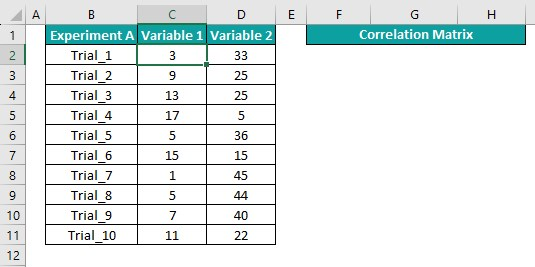To determine the degree of the linear correlation between the variables, we can calculate the correlation matrix to obtain the correlation coefficient for the variables and perform the required analysis. Assume the target cell is cell F2.

Step 1: Select DataData Analysis to open the Data Analysis window.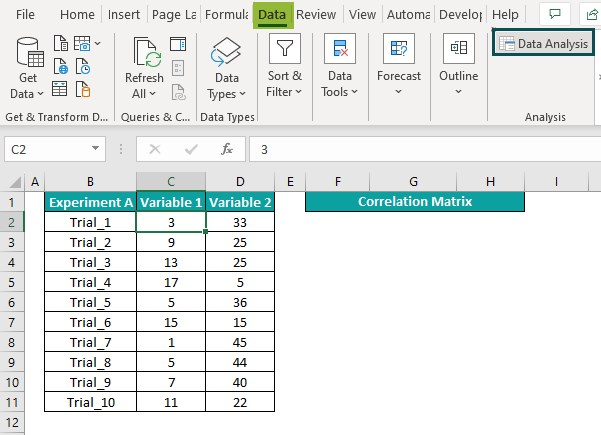Step 2: The Data Analysis window lists the analysis tools, from which we must choose Correlation.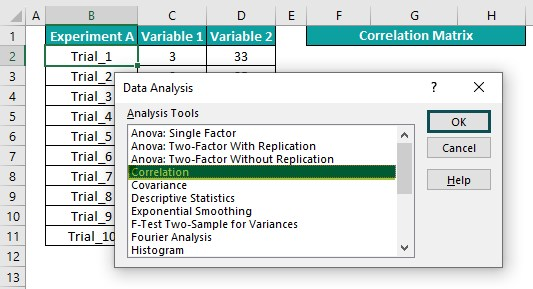And clicking OK will open the Correlation window.

Step 3: Update the input range, Grouped By and label fields, and the target cell reference in the Output Range field in the Correlation window, as shown below.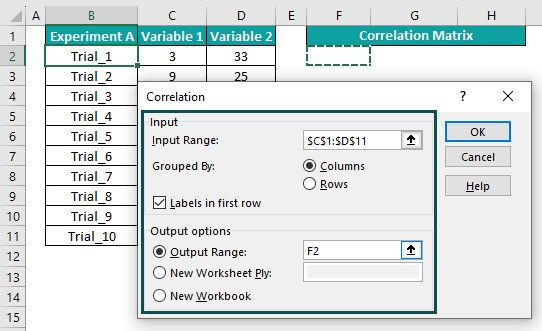And clicking OK will give the following correlation matrix in the target cell.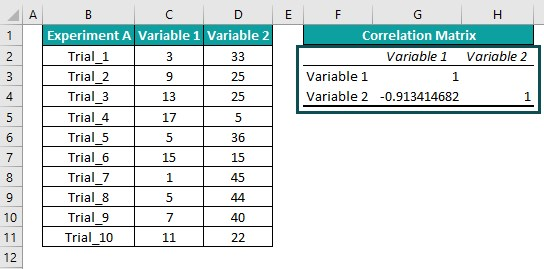The outcome shows that the correlation matrix is negative, with the correlation coefficient for variables 1 and 2 being negative, -0.913414682.

The negative value is close to -1. Thus, it implies that the correlation between the variables is strongly negative, and they move in opposite directions at similar rates.

2. Why is Correlation Matrix in Excel used?

The Correlation Matrix in Excel is used for the following applications:
For summarizing a massive dataset to analyze the correlations patterns.
As inputs in analyses such as the Exploratory Factor review and Structural Equation models.

3. How to detect multicollinearity in Correlation Matrix in Excel?

We can detect multicollinearity in Correlation Matrix in Excel by checking the correlation coefficients for the variable pairs. If the absolute correlation coefficient of two or more variables exceeds 0.7, it indicates multicollinearity in the correlation matrix.